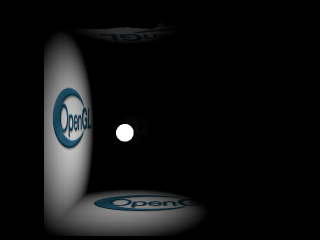# Per Pixel Attenuation## Description:

This program displays a cube consisting simply of six quads. A light, using per-pixel attenuation, bounces inside the cube. The attenuation is calculated in four ways.

For the first three methods, the attenuation equation used is:

`Attenuation=1-(d^2)/(r^2)`

where:

 d is the distance from the point to the light r is the light's radius

The fourth method uses a Gaussian equation:

`Attenuation=exp(-k*d^2)`

#### Method 1

This is the method used by my "Per Pixel Lighting" project. It uses two textures storing the attenuation values, and computes 1-(Tex1+Tex2) in the register combiners.

#### Method 2

Using PASS_THROUGH_NV, part of NV_texture_shader, allows the texture coordinates passed to a texture unit to be interpolated, clamped to [0,1] and made available to the register combiners. The attenuation function is then calculated in the combiners.

Advantages: No textures used, Only 1 TMU used, Fastest method

#### Method 3

This method is much like method 1, but uses a 3d texture instead of 2 textures. This saves a TMU.

#### Method 4

Gaussian attenuation is implemented similarly to method 1. It uses two textures to compute the attenuation, but using a simpler equation. The Gaussian function is separable by multiplication rather than by addition, so all that is needed to calculate it is a simple modulation of two textures. In this demo, this is done with register combiners for similarity to the other methods, but this can easily be calculated without.

Advantages: Low hardware requirements (only multitexture required)

## Requirements:

EXT_texture_edge_clamp
ARB_multitexture with at least 3 texture units
NV_vertex_program
NV_register_combiners

EXT_texture3D